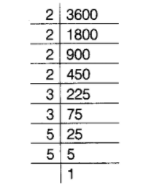# By what smallest number should 3600 be multiplied,

Question:

By what smallest number should 3600 be multiplied, so that the quotient is a perfect cube. Also, find the cube root of the quotient.

Solution:

Prime factors of 3600 = 2x2x2x2x3x3x5x5

Grouping the factors into triplets of equal factors, we get

$3600=\underline{2 \times 2 \times 2 \times 2 \times 3 \times 3 \times 5 \times 5}$We know that, if a number is to be a perfect cube, then each of its prime factors must occur thrice.

We find that 2 occurs once 3 and 5 occurs twice only.

Hence, the smallest number, by which the given number must be multiplied in order that the product is a perfect cube $=2 \times 2 \times 3 \times 5=60$

Also, product $=3600 \times 60=216000$

Now, arranging into triplets of equal prime factors, we have

$216000=\underline{2 \times 2 \times 2} \times 2 \times 2 \times 2 \times \underline{3} \times 3 \times 3 \times 5 \times 5 \times 5$

Taking one factor from each triplets, we get

$\sqrt{216000}=2 \times 2 \times 3 \times 5=60$